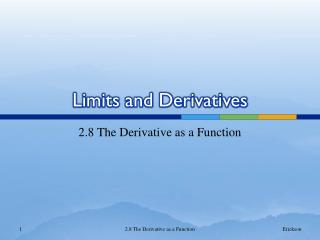DownloadDownload PresentationLimits and Derivatives

# Limits and Derivatives

Download Presentation## Limits and Derivatives

- - - - - - - - - - - - - - - - - - - - - - - - - - - E N D - - - - - - - - - - - - - - - - - - - - - - - - - - -
##### Presentation Transcript

1. Limits and Derivatives 2.8 The Derivative as a Function 2.8 The Derivative as a Function

2. Derivative Last class, we considered the derivative of a function f at a fixed number a, 2.8 The Derivative as a Function

3. Derivative Today, we want to let avary in order to create a function. To do so we replace ain our previous equation with x and get the derivative of f defined by 2.8 The Derivative as a Function

4. Interpretation of the Derivative • Remember, the value of f ′(x) can be interpreted geometrically as the slope of the tangent line to the graph of the original function f at the point (x, f (x)). • We can use the graph of the function to sketch the derivative. 2.8 The Derivative as a Function

5. Example 1 • The graph of a function f is given below. Use it to sketch the graph of the derivative f '. 2.8 The Derivative as a Function

6. Example 1 • Solution 2.8 The Derivative as a Function

7. Try these on your own… • Sketch the Derivative 2.8 The Derivative as a Function

8. Example 2 • Find f ′ and state the domain of f ′ if 2.8 The Derivative as a Function

9. Other Notations All of the following mean the derivative of the function with respect to x. Newton’s Dot Notation: Leibniz: Lagrange: Euler: Prime Notation: 2.8 The Derivative as a Function

10. Other Notations • If we want to indicate the value of a derivative dy/dx in Leibniz notation at a specific number a, we use the notation which is a synonym for f ′(a). 2.8 The Derivative as a Function

11. Other Derivatives • Function – Position x • First derivative – Velocity • Second derivative – Acceleration • Third derivative - Jerk 2.8 The Derivative as a Function

12. Finding the Second Derivative 2.8 The Derivative as a Function

13. Example 3 • Find the first and second derivatives of the following function: 2.8 The Derivative as a Function

14. Book Example – Page 162 #1 • Use the graph to estimate the value of each derivative. Sketch the graph of f’. 2.8 The Derivative as a Function

15. Book Example – Page 162 #2 • Use the graph to estimate the value of each derivative. Sketch the graph of f’. 2.8 The Derivative as a Function

16. Book Example – Page 162 # 6, 8, & 10 • Trace or copy the graph of the given function f. Assuming that the axis have equal scales, sketch the graph of f’ below it. 2.8 The Derivative as a Function

17. Example • Find the derivative of the function using the definition of its derivative. State the domain of the function and the domain of its derivative. 2.8 The Derivative as a Function

18. Book Example – Page 163 #33 • If , find f(x). • Check to see that your answer in (a) is reasonable by comparing the graphs of f and f. 2.8 The Derivative as a Function

19. Book Example – Page 164 #35 • The unemployment rate U(t) varies with time. The table from the Bureau of Statistics gives the percentage of unemployed in the US labor force from 1999 to 2008. • What is the meaning of U(t)? What are its units? • Construct a table of values for U(t). 2.8 The Derivative as a Function

20. Book Example – Page 164 #45 • The figure shows the graphs of three functions. One is the position function of a car, one is the velocity function of a car, and one is acceleration of the car. Identify each curve and explain your choices. 2.8 The Derivative as a Function

21. What’s next? • So far we have: • Interpreted derivatives as slopes and rates of changes • Estimated derivatives of functions given by tables of values • Graphed derivatives of functions that are defined graphically • Used the definition of derivative to calculate the derivatives of functions defined by formulas. • Next time we will develop rules for finding the derivatives without having to use the definition directly. 2.8 The Derivative as a Function